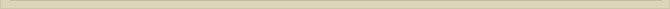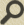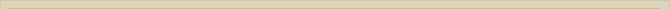## The moving electron and magnetic energy

When you are interested in physics you must read “Unbelievable“!

The classical radius or Compton radius (Rc) of an electron is calculated means of the Compton-equation: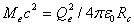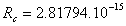meter.

With Me the rest mass of the electron, Qe the elementary charge of an electron.

The equation for the magnetic energy of a charged (Qe) bulb (Rc) moving with a relative speed Ve, is: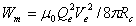When substituting the mass equivalence, Me, of the Compton-equation in the formula for the magnetic energy Wm and considering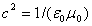, we get: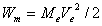So when we assume the electron has the radius Rc, derived with the Compton-equation, the magnetic energy of the moving electron presents energy equal to the kinetic energy of that same electron.

A moving “pure electrostatic mass” presents magnetic energy according to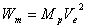, while the magnetic energy of a moving electron with the Compton-radius Rc has a magnetic energy of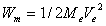.

What causes the difference?

Considering the Compton-equationand the electrostatic energy of a charged bulb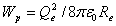, we observe that the difference between the formulas is a factor two. The Compton-equation “stores” twice as much energy as a charged bulb with the same charge and radius. This difference explains exactly the difference between the equations for the magnetic energy, for the moving charged bulb, andfor the moving electron with the Compton-radius.

The Compton-equation “stores” twice as much energy as the formula for a charged bulb. We know that apart from a charge, the electron also has a spin. The magnetic spin of the electron is not considered in the Compton-equation.

In the previous chapter “The Electron” the total energy of an electron Wt, at rest, is presented the formula:In this presentation of the total energy of an electron at rest, half the energy is presented electrostatic energy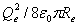, consistent with the energy of a charged bulb, and the other half of the energy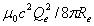, the magnetic spin energy of the electron.

Calculating the radius with this equation, the radius Re of an electron becomesmeter: exact the Compton radius.

When we consider half the intrinsic energy of the electron at rest is presented electrostatic energy and the other half the magnetic spin energy, the magnetic energy of a moving electron will be: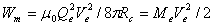When half of the intrinsic energy of the mass of the electron is presented the electrostatic energy and the other half the magnetic spin energy, the calculated magnetic energy of the moving electron is equal to the kinetic energy of that electron.

Because the kinetic energy of an electron is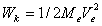and at the same time the magnetic energy (Wm) is also, the kinetic energy of the electron must be the same energy as the magnetic energy. Otherwise the conservation law for energy is violated every time an electron is accelerated or slowed down.

Next chapter: The Electromagnetic Mass